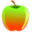CBEST Test Prep

# CBEST Math Test Tips - Page one.

### Mental Math Tips

The following section is for those who have a fear of math, are not very good at it, think they are not very good at it, or those who just want alternative methods for solving problems. This section does include methods for fractions, decimals, and percents.

Mental Math Skills Needed for CBEST Test

Mental math will make you understand what numbers are all about. They are nothing to fear or avoid. They will help you logically make guesses on any multiple choice test. This is a way of doing math that is radically different than how it is taught. If you already know how to do the following using other methods, this will still help you become a whiz at math and be very comfortable with numbers.

Know your basic math skills. This includes adding, subtracting, multiplying and dividing numbers between 0 and 10. Know how to these small numbers relate to large numbers.

Be able to divide rapidly by 2, or in other words, cutting something in half. It is easier than you think if you split numbers up.
Example: What is 457 ÷ 2?
Think: 400 ÷ 2 = 200, 50 ÷ 2 = 25, 7 ÷ 2 = 3.5, so the answer is 200 + 25 + 3.5 or 228.5. Easy!
Try cutting 569 in half.
Practice this concept!

2 + 4 and 5 + 7 are things you can rapidly do in your head or should be able to. Then logically take it a step further. If 5 + 7 = 12, then how does that relate to 15 + 7 ? It is simply 2 more than the next 10 or 22. Can you see how knowing 5 + 7 = 12 will help you do 77 + 5 ?  77 + 5 becomes almost automatic. How about 2 + 4 ? It is related to 22 + 24 and 52 + 64. Practice this concept!

Let's take 7 + 8 for example. It equals 15. That is 5 more than the next 10. We can now rapidly do 227 + 8. Perhaps before you would have shied away from that because 227 looks like a large number. It is simply 235.

Know something about adding 0. 6 + 0 = 6, 10 + 6 = 16, 20 + 17 = 37, 40 + 22 = 62, 56 + 30 = 86. You should be able to logically get these answers with little time. Practice this concept!

Know how to get a total of 100 from 2 numbers.
Example: 69 + 31 = 100, 43 + 57 = 100, etc.

Be able to double numbers in your head, or get a close approximation. If 2 x 14 = 28, then 4 x 14 must be 56, and so on. 10 x 13 = 130, 20 x 13 = 260, 40 x 13 = 520, etc. Practice this concept!

Split numbers up to add them.
Examples:
45 + 56 = 40 + 50 + 5 + 6 = 101.
67 + 14 = 60 + 10 + 7 + 4 = 11
Try it this way: 59 + 23 = (50 + 20) + (9 + 3) = (70 + 12) = 82

Similar for large numbers.
Examples:
345 + 654 = (300 + 600) + ( 40 + 50) + (5 + 4) = 900 + 90 + 9 = 999.
Practice this concept!

Know the difference between numbers and 100.
Example, know 100 - 56 = 44, 100 - 36 = 64, 100 - 37 = 63, 100 - 25 = 75, etc. Practice this concept!

Know how multiples of 10 are related. 2 + 4 is really the same as 20 + 40, and 200 + 400. They will all start with a 6. Everyone can do 200 + 400 = 600 in their head, just like we can 30 + 60 in our heads. How does this help us? It helps us set boundaries. Practice this concept!

To take it a step further, we need to understand the boundaries of some calculations. Suppose we have 27 + 38.
We should realize that 27 + 38 is related to 7 + 8 in the fact that it will end with a 5. Look at the number 27. It is between 20 and 30. The number 38 is between 30 and 40. If we add the lower boundaries of each number we get 50 ( 20 + 30). If we add the upper boundaries of each number, we get 70 ( 30 + 40). We now know the answer lies between 50 and 70. Given the fact that we know the answer lies between 50 and 70, and it ends in a 5, we have 2 choices: 55 or 65. We should also use logic to tell us the answer of 55 is too low. How ? Think 27 + 38 is larger than 27 + 30 or even 20 + 38. Therefore the answer must be more than 57 or 58.

## More CBEST Test Prep Resources

CBEST Test Tips - Overall tips to take and pass the CBEST Test.

CBEST Math Tips - Read our tips to pass the math section of the CBEST Test.

Essay Writing Tips for the CBEST - Get our CBEST essay writing guide here.